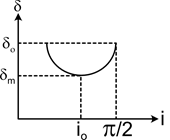In the diagram shown, a plot between δ (angle of deviation) versus i (angle of incidence) for a triangular prism is given. From the observed plot, some conclusion can be drawn. Mark out the correct conclusion.

# In the diagram shown, a plot between $\delta$ (angle of deviation) versus i (angle of incidence) for a triangular prism is given. From the observed plot, some conclusion can be drawn. Mark out the correct conclusion.1. A

The range of deviation for which two angles of incidence are possible for same deviation is ${\delta }_{0}-{\delta }_{m}$

2. B

The curve is asymmetrical about ${\mathrm{i}}_{0}$

3. C

For a given $\delta$, i is unique

4. D

Both 1 and 2 are correct.

Register to Get Free Mock Test and Study Material

+91

Verify OTP Code (required)

### Solution:

The graph is asymmetrical about ${\mathrm{i}}_{0}$ the section to the left of ${\mathrm{i}}_{0}$ being steeper than the section to the right.Register to Get Free Mock Test and Study Material

+91

Verify OTP Code (required)